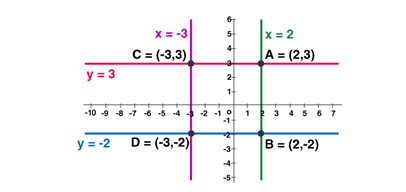• +91 9971497814
• info@interviewmaterial.com

# RD Chapter 23- The Straight Lines Ex-23.2 Interview Questions Answers

### Related Subjects

Question 1 : Find the equation of the parallel to x–axis and passing through (3, –5).

Given: A line which isparallel to x–axis and passing through (3, –5)

By using the formula,

The equation of line:[y – y1 = m(x – x1)]

We know that theparallel lines have equal slopes

And, the slope ofx–axis is always 0

Then

The slope of line, m =0

Coordinates of lineare (x1, y1) = (3, –5)

The equation of line =y – y1 = m(x – x1)

Now, substitute thevalues, we get

y – (– 5) = 0(x – 3)

y + 5 = 0

The equation of lineis y + 5 = 0

Question 2 : Find the equation of the line perpendicular to x–axis and having intercept – 2 on x–axis.

Given: A line which isperpendicular to x–axis and having intercept –2

By using the formula,

The equation of line:[y – y1 = m(x – x1)]

We know that, the lineis perpendicular to the x–axis, then x is 0 and y is –1.

The slope of line is,m = y/x

= -1/0

It is given thatx–intercept is –2, so, y is 0.

Coordinates of lineare (x1, y1) = (–2, 0)

The equation of line =y – y1 = m(x – x1)

Now, substitute thevalues, we get

y – 0= (-1/0) (x – (– 2))

x + 2 = 0

The equation of lineis x + 2 = 0

Question 3 : Find the equation of the line parallel to x–axis and having intercept – 2 on y – axis.

Given: A line which isparallel to x–axis and having intercept –2 on y – axis

By using the formula,

The equation of line:[y – y1 = m(x – x1)]

The parallel lineshave equal slopes,

And, the slope ofx–axis is always 0

Then

The slope of line, m =0

It is given thatintercept is –2, on y – axis then

Coordinates of lineare (x1, y1) = (0, – 2)

The equation of lineis y – y1 = m(x – x1)

Now, substitute thevalues, we get

y – (– 2) = 0 (x – 0)

y + 2 = 0

The equation of lineis y + 2 = 0

Question 4 : Draw the lines x = –3, x = 2, y = –2, y = 3 and write the coordinates of the vertices of the square so formed.

Given: x = –3, x = 2,y = –2 and y = 3The Coordinates ofthe square are: A(2, 3), B(2, –2), C(–3, 3), and D(–3, –2).

Question 5 : Find the equations of the straight lines which pass through (4, 3) and are respectively parallel and perpendicular to the x–axis.

Given: A line which isperpendicular and parallel to x–axis respectively and passing through (4, 3)

By using the formula,

The equation of line:[y – y1 = m(x – x1)]

Let us consider,

Case 1: When Line isparallel to x–axis

The parallel lineshave equal slopes,

And, the slope ofx–axis is always 0, then

The slope of line, m =0

Coordinates of lineare (x1, y1) = (4, 3)

The equation of lineis y – y1 = m(x – x1)

Now substitute thevalues, we get

y – (3) = 0(x – 4)

y – 3 = 0

Case 2: When line isperpendicular to x–axis

The line isperpendicular to the x–axis, then x is 0 and y is – 1.

The slope of the lineis, m = y/x

= -1/0

Coordinates of lineare (x1, y1) = (4, 3)

The equation of line =y – y1 = m(x – x1)

Now substitute the values,we get

y – 3 = (-1/0) (x – 4)

x = 4

The equation of linewhen it is parallel to x – axis is y = 3 and it is perpendicular is x = 4.

Todays Deals### RD Chapter 23- The Straight Lines Ex-23.2 Contributorskrishan

Name:
Email:

# Latest News# 9000 interview questions in different categories